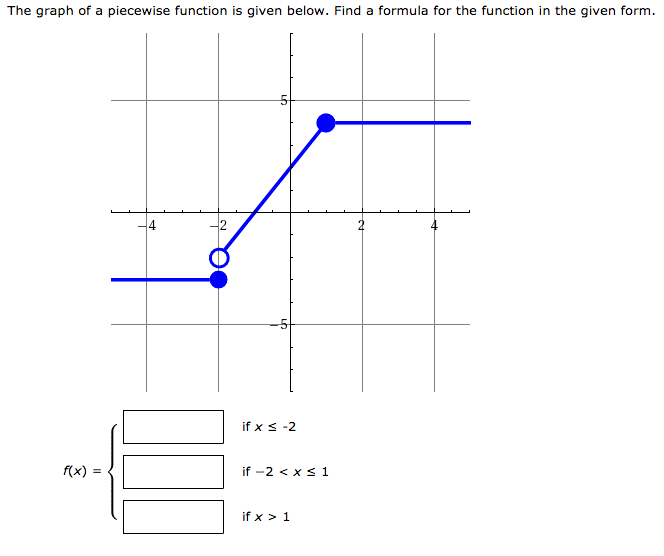# Filling in piecewise function from given graph

• Niaboc67
In summary, the conversation is about understanding a question related to piecewise functions and finding the equation that passes through two given points. The speaker suggests starting with the easier parts of the question and mentions that the concept of functions is typically covered in the first chapter of calculus books.

## Homework Statement## The Attempt at a Solution

Not sure what to do here. I was thinking maybe the y y1 = m(x x1)? I am having trouble understanding this question. I know what piecewise functions are but filling this in is proving difficult.

Niaboc67 said:

## The Attempt at a Solution

Not sure what to do here. I was thinking maybe the y y1 = m(x x1)? I am having trouble understanding this question. I know what piecewise functions are but filling this in is proving difficult.
Start with x<=-2. What is the value of y at x=-3? What is its value at x=-2. What equation of the form you suggest passes through those two points?

Lift your morale by doing the easy parts first. It is pretty clear on the graph what x and f(x) (which maybe you think of as the 'y axis') are meant to be at the points represented by blobs. You can't have any difficulty then in knowing what f(x) is when x ≥ 1 surely?

Edit :I am quite often surprised when students come here asking questions about what is in the first section of Chapter 1 of every book on the subject. This looks like the explanation of what is meant by 'a function' which is typically in that chapter of every book on calculus. 50:1 a very similar example is at the end of that first section in your book.

Last edited: